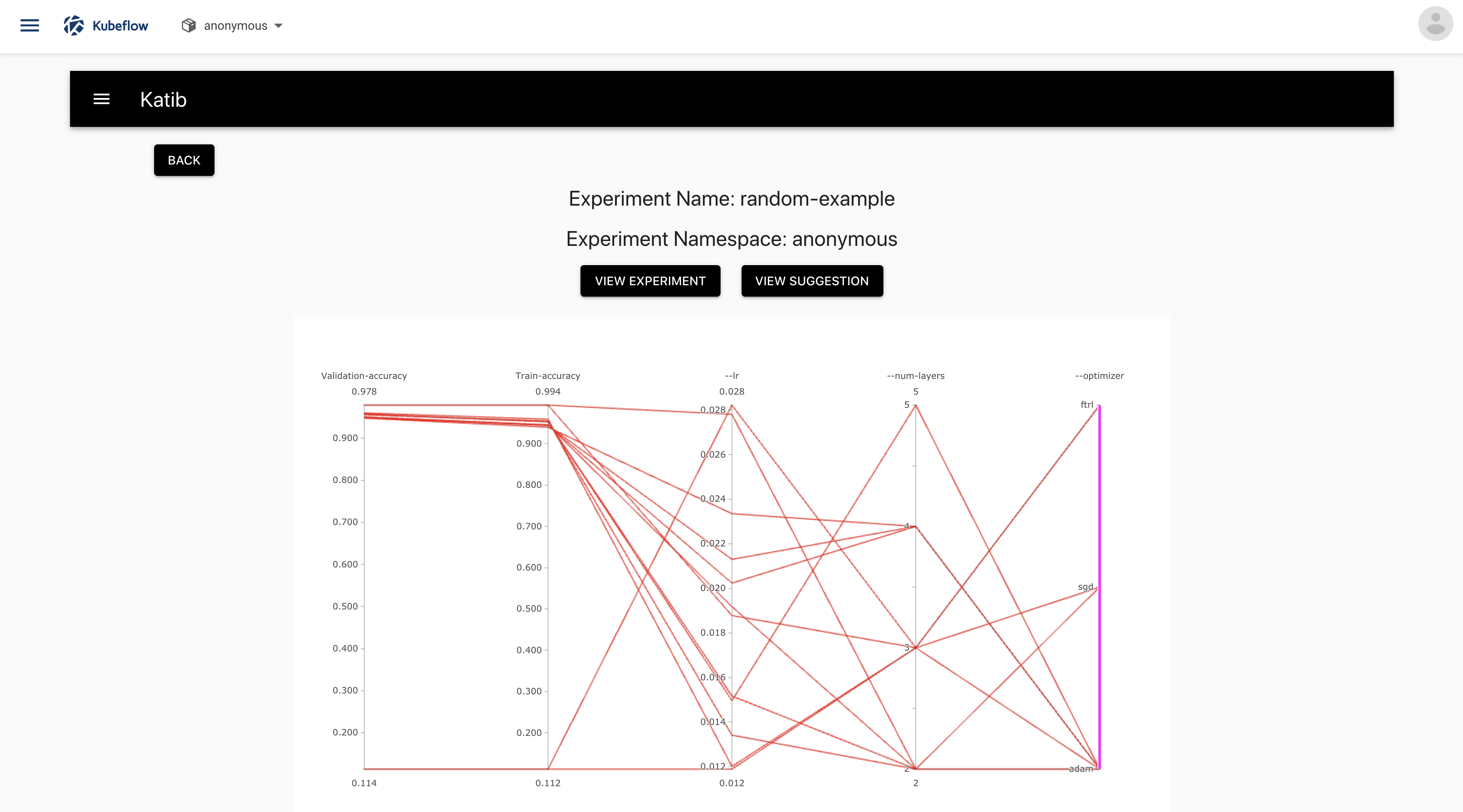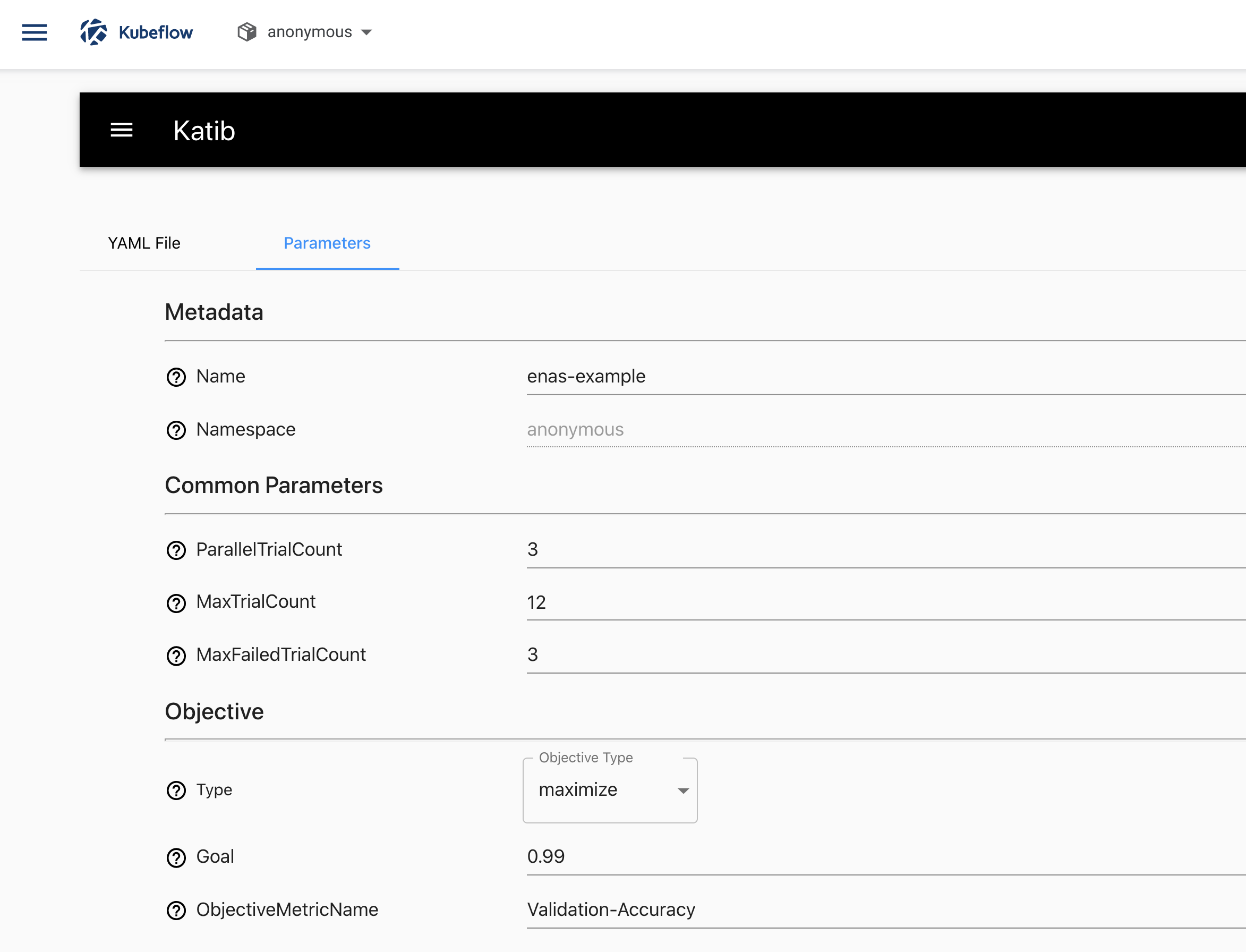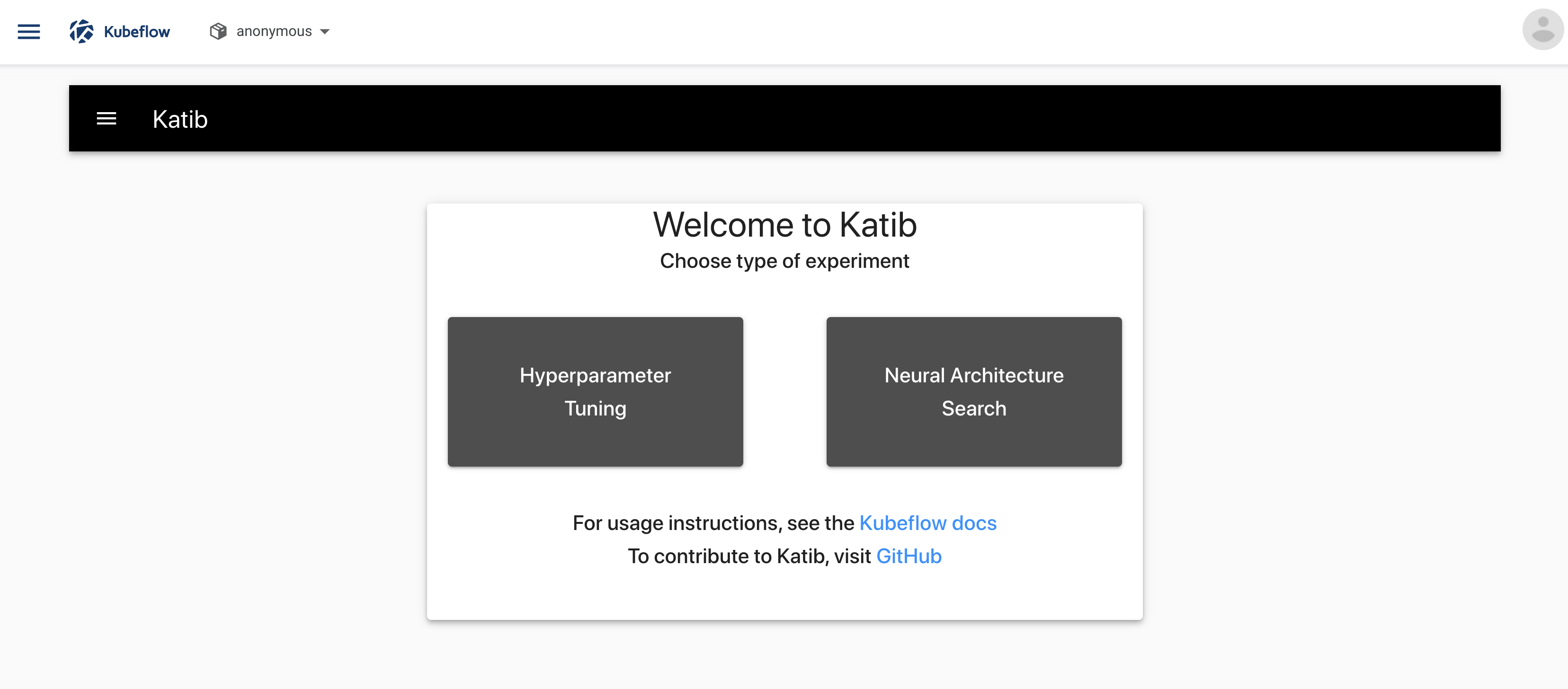# Introduction to Katib

Overview of Katib for hyperparameter tuning and neural architecture search

Use Katib for automated tuning of your machine learning (ML) model’s hyperparameters and architecture.

This page introduces the concepts of hyperparameter tuning, neural architecture search, and the Katib system as a component of Kubeflow.

## Hyperparameters and hyperparameter tuning

Hyperparameters are the variables that control the model training process. For example:

• Learning rate.
• Number of layers in a neural network.
• Number of nodes in each layer.

Hyperparameter values are not learned. In other words, in contrast to the node weights and other training parameters, the model training process does not adjust the hyperparameter values.

Hyperparameter tuning is the process of optimizing the hyperparameter values to maximize the predictive accuracy of the model. If you don’t use Katib or a similar system for hyperparameter tuning, you need to run many training jobs yourself, manually adjusting the hyperparameters to find the optimal values.

Automated hyperparameter tuning works by optimizing a target variable, also called the objective metric, that you specify in the configuration for the hyperparameter tuning job. A common metric is the model’s accuracy in the validation pass of the training job (validation-accuracy). You also specify whether you want the hyperparameter tuning job to maximize or minimize the metric.

For example, the following graph from Katib shows the level of validation accuracy for various combinations of hyperparameter values (learning rate, number of layers, and optimizer):(To run the example that produced this graph, follow the getting-started guide.)

Katib runs several training jobs (known as trials) within each hyperparameter tuning job (experiment). Each trial tests a different set of hyperparameter configurations. At the end of the experiment, Katib outputs the optimized values for the hyperparameters.

In addition to hyperparameter tuning, Katib offers a neural architecture search (NAS) feature. You can use the NAS to design your artificial neural network, with a goal of maximizing the predictive accuracy and performance of your model.

NAS is closely related to hyperparameter tuning. Both are subsets of automated machine learning (AutoML). While hyperparameter tuning optimizes the model’s hyperparameters, a NAS system optimizes the model’s structure, node weights, and hyperparameters.

NAS technology in general uses various techniques to find the optimal neural network design.

You can submit Katib jobs from the command line or from the UI. (Read more about the Katib interfaces later on this page.) The following screenshot shows part of the form for submitting a NAS job from the Katib UI:## The Katib project

Katib is a Kubernetes-based system for hyperparameter tuning and neural architecture search. Katib supports a number of ML frameworks, including TensorFlow, MXNet, PyTorch, XGBoost, and others.

The Katib project is open source. The developer guide is a good starting point for developers who want to contribute to the project.

## Katib interfaces

You can use the following interfaces to interact with Katib:

• A web UI that you can use to submit experiments and to monitor your results. See the getting-started guide for information on how to access the UI. The Katib home page within Kubeflow looks like this:• A REST API. See the API reference on GitHub.

• Command-line interfaces (CLIs):

• Kfctl is the Kubeflow CLI that you can use to install and configure Kubeflow. Read about kfctl in the guide to configuring Kubeflow.

• The Kubernetes CLI, kubectl, is useful for running commands against your Kubeflow cluster. Read about kubectl in the Kubernetes documentation.

• Katib SDK. See the Katib SDK documentation on GitHub.

## Katib concepts

This section describes the terms used in Katib.

### Experiment

An experiment is a single tuning run, also called an optimization run.

You specify configuration settings to define the experiment. The following are the main configurations:

• Objective: What you want to optimize. This is the objective metric, also called the target variable. A common metric is the model’s accuracy in the validation pass of the training job (validation-accuracy). You also specify whether you want the hyperparameter tuning job to maximize or minimize the metric.

• Search space: The set of all possible hyperparameter values that the hyperparameter tuning job should consider for optimization, and the constraints for each hyperparameter. Other names for search space include feasible set and solution space. For example, you may provide the names of the hyperparameters that you want to optimize. For each hyperparameter, you may provide a minimum and maximum value or a list of allowable values.

• Search algorithm: The algorithm to use when searching for the optimal hyperparameter values.

For details of how to define your experiment, see the guide to running an experiment.

### Suggestion

A suggestion is a set of hyperparameter values that the hyperparameter tuning process has proposed. Katib creates a trial to evaluate the suggested set of values.

### Trial

A trial is one iteration of the hyperparameter tuning process. A trial corresponds to one worker job instance with a list of parameter assignments. The list of parameter assignments corresponds to a suggestion.

Each experiment runs several trials. The experiment runs the trials until it reaches either the objective or the configured maximum number of trials.

### Worker job

The worker job is the process that runs to evaluate a trial and calculate its objective value.

The worker job can be one of the following types:

By offering the above worker job types, Katib supports multiple ML frameworks.

## Next steps

Follow the getting-started guide to set up Katib and run some hyperparameter tuning examples.